# Data with Data Structures with Structures Java and

• Slides: 43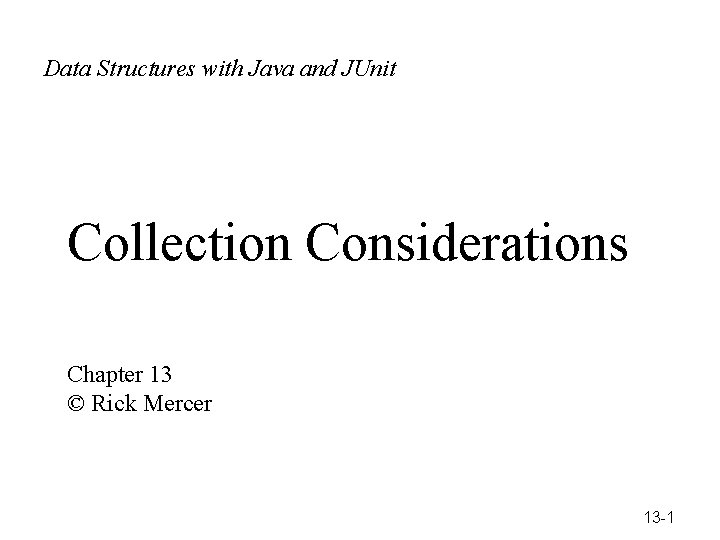Data with Data Structures with. Structures Java and JUnit Ja ©Rick Mercer Collection Considerations Chapter 13 © Rick Mercer 13 -1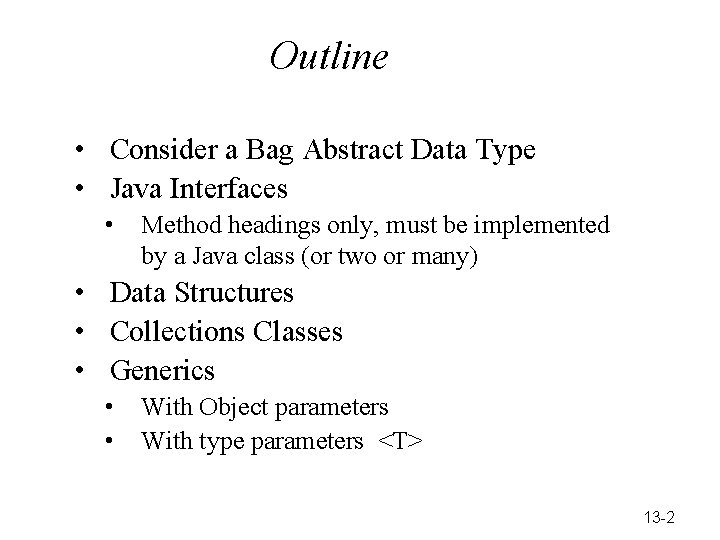Outline • Consider a Bag Abstract Data Type • Java Interfaces • Method headings only, must be implemented by a Java class (or two or many) • Data Structures • Collections Classes • Generics • • With Object parameters With type parameters <T> 13 -2Some Definitions Abstract Data Type (ADT) A set of data values and associated operations that are precisely specified independent of any particular implementation. Bag, Set, List, Stack, Queue, Map Collection Class A Java language construct for encapsulating the data and operations Array. List, Linked. List, Stack, Tree. Set, Hash. Map Data Structure An organization of information usually in memory arrays, linked structure, binary trees, hash tables 13 -3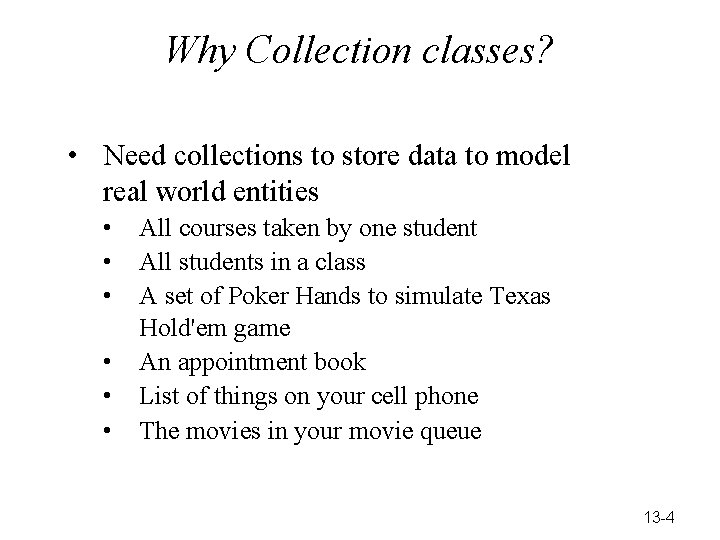Why Collection classes? • Need collections to store data to model real world entities • • • All courses taken by one student All students in a class A set of Poker Hands to simulate Texas Hold'em game An appointment book List of things on your cell phone The movies in your movie queue 13 -4Common Methods • Collection classes often have methods for performing operations such as these • • • Adding an object to the collection of objects Removing an object from the collection Getting a reference to a particular object find • then you can send messages to the object while it is till in the collection. Program do this a lot • • Retrieving certain objects such as the most recently pushed (Stack) or least recently enqueued (Queue) Arranging objects in a certain order sorting • The most basic collection is a Bag 13 -5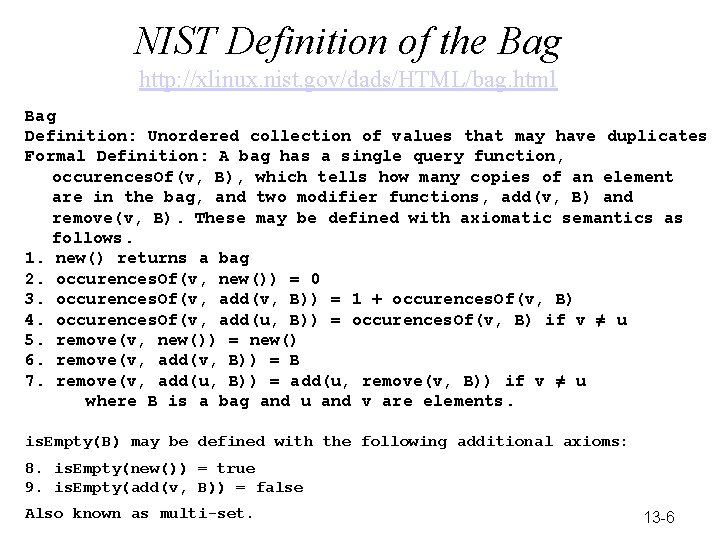NIST Definition of the Bag http: //xlinux. nist. gov/dads/HTML/bag. html Bag Definition: Unordered collection of values that may have duplicates Formal Definition: A bag has a single query function, occurences. Of(v, B), which tells how many copies of an element are in the bag, and two modifier functions, add(v, B) and remove(v, B). These may be defined with axiomatic semantics as follows. 1. new() returns a bag 2. occurences. Of(v, new()) = 0 3. occurences. Of(v, add(v, B)) = 1 + occurences. Of(v, B) 4. occurences. Of(v, add(u, B)) = occurences. Of(v, B) if v ≠ u 5. remove(v, new()) = new() 6. remove(v, add(v, B)) = B 7. remove(v, add(u, B)) = add(u, remove(v, B)) if v ≠ u where B is a bag and u and v are elements. is. Empty(B) may be defined with the following additional axioms: 8. is. Empty(new()) = true 9. is. Empty(add(v, B)) = false Also known as multi-set. 13 -6We use Java interfaces rather than axiomatic expressions /** * This interface specifies the methods for a Bag ADT. A bag * is also known as a multi-set because bags are like a set * with duplicate elements allowed. */ public interface Bag { // Return true if there are no elements in this Bag. public boolean is. Empty(); // Add a v to this collection. public void add(Object v); // Return how often the value v exists in this String. Bag. public int occurences. Of(Object v); // If an element that equals v exists, remove one // occurrence of it from this Bag and return true. // If occurences. Of(v) == 0, simply return false. public boolean remove(Object v); } 13 -7The Java interface construct • A Java interface describes a set of methods: • no constructors • no instance variables • The interface must be implemented by some class. • Over 1, 000 java classes implement one or more interfaces • Consider a simple interface 13 -8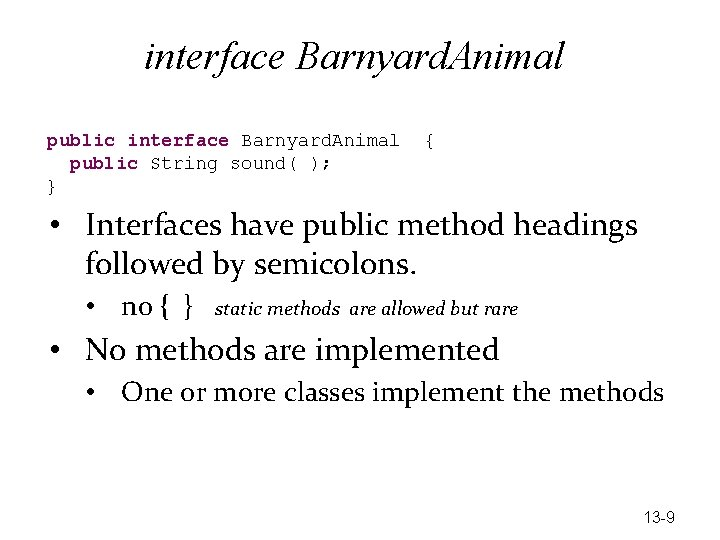interface Barnyard. Animal public String sound( ); } { • Interfaces have public method headings followed by semicolons. • no { } static methods are allowed but rare • No methods are implemented • One or more classes implement the methods 13 -9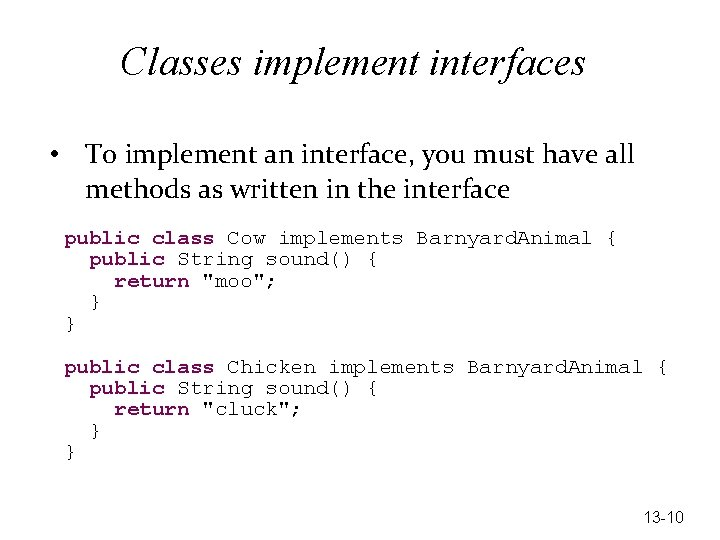Classes implement interfaces • To implement an interface, you must have all methods as written in the interface public class Cow implements Barnyard. Animal { public String sound() { return "moo"; } } public class Chicken implements Barnyard. Animal { public String sound() { return "cluck"; } } 13 -10Cow and Chicken are also known as a Barnyard. Animal a. Cow = new Cow(); Barnyard. Animal a. Chicken = new Chicken(); assert. Equals(_______, a. Cow. sound()); assert. Equals(_______, a. Chicken. sound()); • Fill in the blanks so the assertions pass • We can store references to a Cow and a Chicken into reference variable of type Barnyard. Animal 13 -11Comparable interface (less silly) • Can assign an instance of a class that implements an interface to a variable of the interface type Comparable str = new String("abc"); Comparable acct = new Bank. Account("B", 1); Comparable day = new Date(); • A few classes that implement Comparable Big. Decimal Big. Integer Byte. Buffer Character Char. Buffer Charset Collation. Key Date Double. Buffer File Float. Buffer Integer Long. Buffer Object. Stream. Field Short. Buffer String URI • Comparable defines the "natural ordering" When is one object less than or greater than another? 13 -12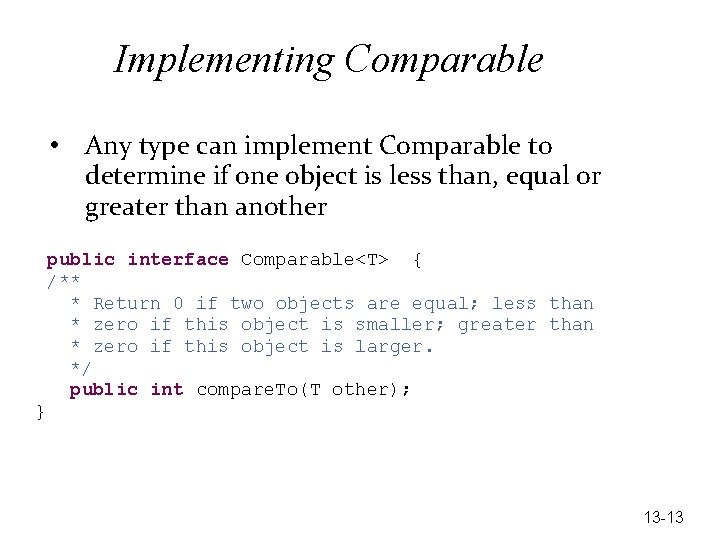Implementing Comparable • Any type can implement Comparable to determine if one object is less than, equal or greater than another public interface Comparable<T> { /** * Return 0 if two objects are equal; less than * zero if this object is smaller; greater than * zero if this object is larger. */ public int compare. To(T other); } 13 -13Let Bank. Account be Comparable public class Bank. Account implements Comparable<Bank. Account> { private String ID; private double balance; public Bank. Account(String ID. . . // stuff deleted } guarantee this class has a compare. To Add this method public int compare. To(Bank. Account other) { // Must complete this method or else it's an error. // Compare by ID, might as well use String's compare. To return get. ID(). compare. To(other. get. ID()); } 13 -14A test method—IDs are compared @Test public void test. Compare. To() { Bank. Account a = new Bank. Account("Alice", 543. 21); Bank. Account z = new Bank. Account("Zac", 123. 45); assert. True(a. compare. To(a) == 0); assert. True(z. compare. To(z) == 0); assert. True(a. compare. To(z) < 0); assert. True(z. compare. To(a) > 0); assert. True(a. compare. To(z) <= 0); assert. True(z. compare. To(a) >= 0); assert. True(z. compare. To(a) != 0); assert. True(a. compare. To(z) != 0); } 13 -15Code Demo 28 -Jan 2015 • • Have Bank. Account implement Comparable public class Array. Bag implements Bag Convert Bag to Bag<E> like in lab and the new projects Test that an Illegal. Argument. Exception is actually thrown • Active/Collaborative learning: write tests for remove and the remove method. See handout 13 -16Generic Collections Classes 1) With Object parameters 2) With Java generics using type parameters 13 -17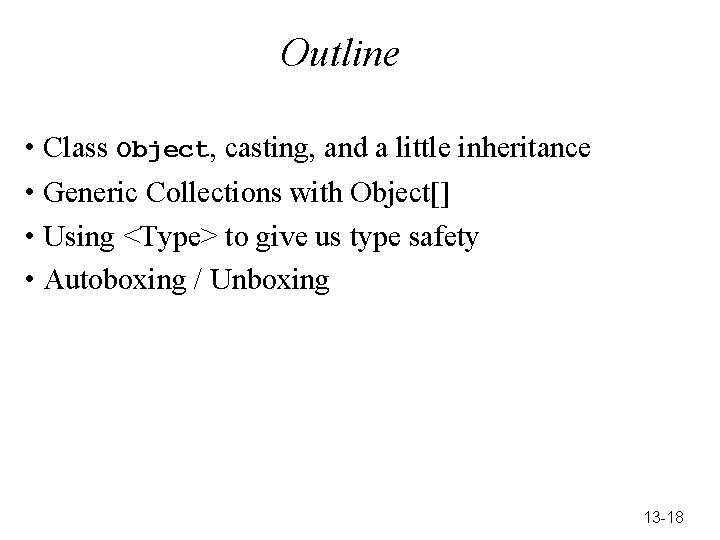Outline • Class Object, casting, and a little inheritance • Generic Collections with Object[] • Using <Type> to give us type safety • Autoboxing / Unboxing 13 -18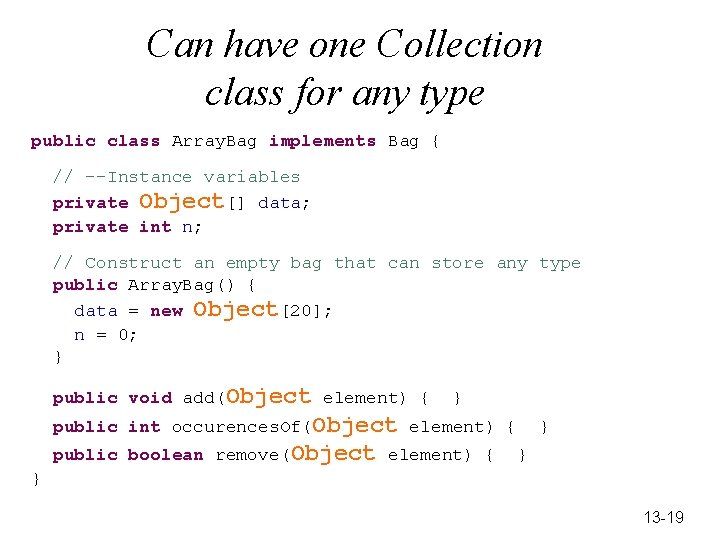Can have one Collection class for any type public class Array. Bag implements Bag { // --Instance variables private Object[] data; private int n; // Construct an empty bag that can store any type public Array. Bag() { data = new Object; n = 0; } public void add(Object element) { } public int occurences. Of(Object element) { public boolean remove(Object element) { } } } 13 -19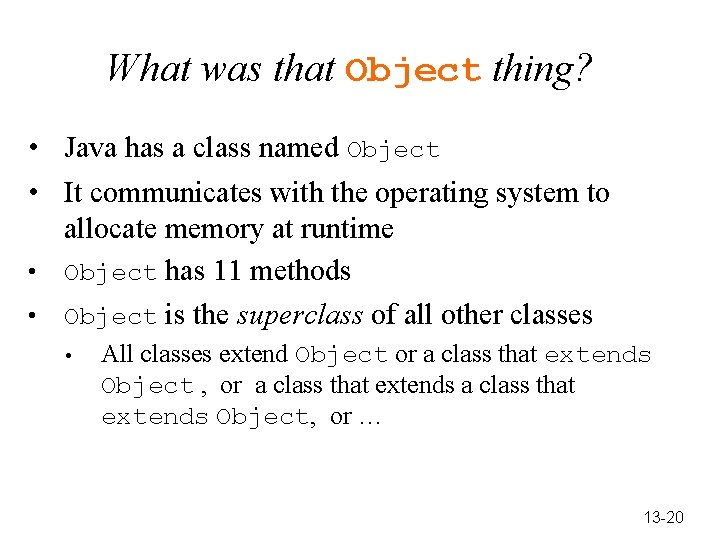What was that Object thing? • Java has a class named Object • It communicates with the operating system to allocate memory at runtime • Object has 11 methods • Object is the superclass of all other classes • All classes extend Object or a class that extends Object , or a class that extends Object, or … 13 -20Empty. Class inherits all 11 methods defined in class Object public class Empty. Class extends Object { // This class inherits Object's 11 methods } // Inherits 11 methods from Object Empty. Class one = new Empty. Class(); Empty. Class two = new Empty. Class(); System. out. println(one. to. String()); System. out. println(one. hash. Code()); System. out. println(one. get. Class()); System. out. println(two. to. String()); System. out. println(two. hash. Code()); System. out. println(one. equals(two)); one = two; System. out. println(one. equals(two)); Output Empty. [email protected] 8 f 763 -4655261 class Empty. [email protected] 763 -4393117 false true 13 -21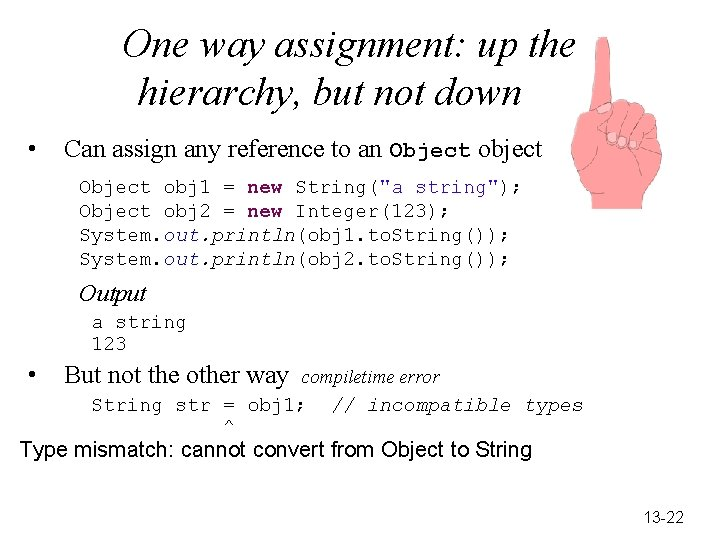One way assignment: up the hierarchy, but not down • Can assign any reference to an Object object Object obj 1 = new String("a string"); Object obj 2 = new Integer(123); System. out. println(obj 1. to. String()); System. out. println(obj 2. to. String()); Output a string 123 • But not the other way compiletime error String str = obj 1; ^ // incompatible types Type mismatch: cannot convert from Object to String 13 -22Tricking the compiler into believing obj 1 is String • Sometimes an explicit cast is needed • Enclose the class name with what you know the class to be in parentheses (String) and place it before the reference to the Object object. str = (String)obj 1; (String) A reference to an Object object 13 -23Example Casts Object obj 1 = new String("A string"); String str = (String) obj 1; Object obj 2 = new Integer(123); Integer an. Int = (Integer) obj 2; Object obj 3 = new Double(123. 45); Double a. Double = (Double) obj 3; Object obj 4 = new Bank. Account(str, a. Double. double. Value()); Double balance = ((Bank. Account) obj 4). get. Balance(); 13 -24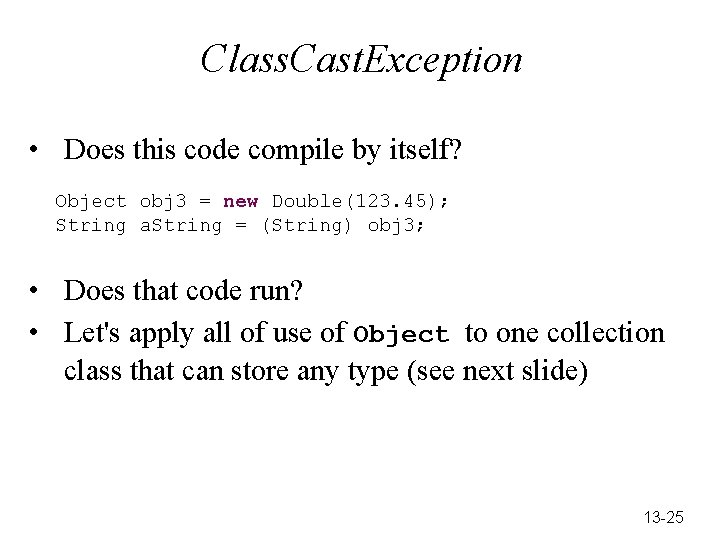Class. Cast. Exception • Does this code compile by itself? Object obj 3 = new Double(123. 45); String a. String = (String) obj 3; • Does that code run? • Let's apply all of use of Object to one collection class that can store any type (see next slide) 13 -25Object can store a reference to type • The Object class allows collections of any type • Use Object[] rather than any one specific type public class Array. Bag implements Bag { private Object[] data; private int n; public Array. Bag() { data = new Object; n = 0; } public void add(Object element) { 13 -26Object as a parameter and a return type • • add has an Object parameter get has an Object and return type • This means that you add or retrieve references to any type object even primitives as we'll see later • This is possible because of inheritance • • can to assign a reference to Object All Java classes extend Object 13 -27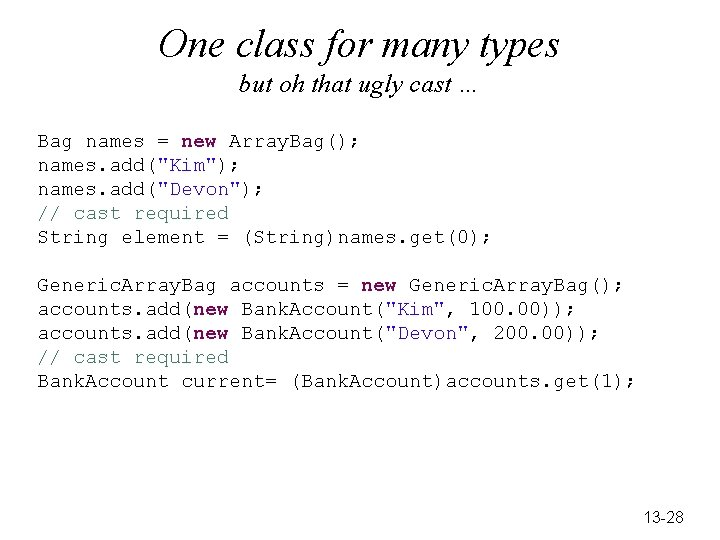One class for many types but oh that ugly cast … Bag names = new Array. Bag(); names. add("Kim"); names. add("Devon"); // cast required String element = (String)names. get(0); Generic. Array. Bag accounts = new Generic. Array. Bag(); accounts. add(new Bank. Account("Kim", 100. 00)); accounts. add(new Bank. Account("Devon", 200. 00)); // cast required Bank. Account current= (Bank. Account)accounts. get(1); 13 -28Generics via Type Parameters <E> A better way to implement collection classes Assumption: Generic. Array. Bag has been implemented using an Object[] instance variable, an Object parameter in add, and an Object return type in get 13 -29One Problem with the old way using Object parameters and return types • • Java "raw" types (no generics) do not check the type This is legal code Bag name = new Array. Bag(); names. add(new Integer(2)); names. add(new Bank. Account("Pat", 2. 00)); names. add(new Gregorian. Calendar(2009, 0, 1)); names. add(1. 23); • So what type do you promise the compiler for these expressions? names. get(0) names. get(1) names. get(2) names. get(3) • • ______ Often get the runtime error Class. Cast. Exception With version 5, Java added a better option: Generics 13 -30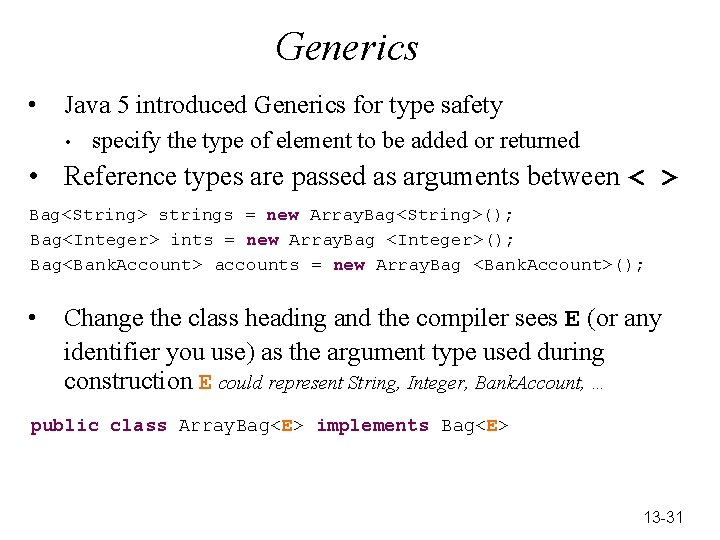Generics • Java 5 introduced Generics for type safety • specify the type of element to be added or returned • Reference types are passed as arguments between < > Bag<String> strings = new Array. Bag<String>(); Bag<Integer> ints = new Array. Bag <Integer>(); Bag<Bank. Account> accounts = new Array. Bag <Bank. Account>(); • Change the class heading and the compiler sees E (or any identifier you use) as the argument type used during construction E could represent String, Integer, Bank. Account, … public class Array. Bag<E> implements Bag<E> 13 -31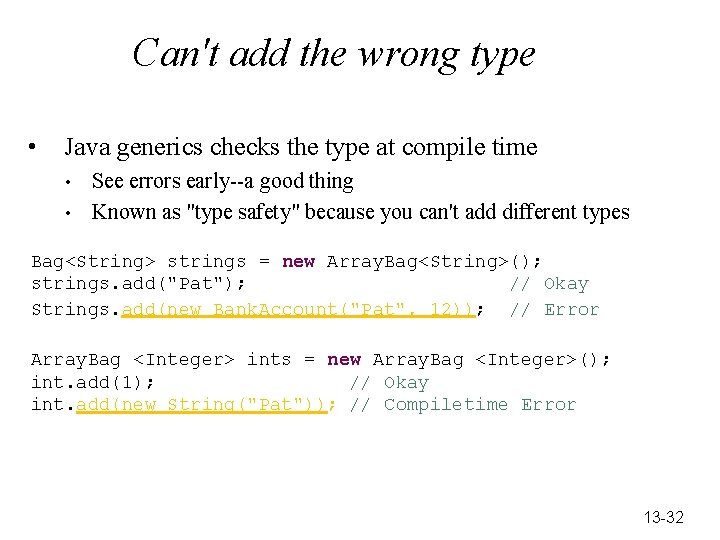Can't add the wrong type • Java generics checks the type at compile time • • See errors early--a good thing Known as "type safety" because you can't add different types Bag<String> strings = new Array. Bag<String>(); strings. add("Pat"); // Okay Strings. add(new Bank. Account("Pat", 12)); // Error Array. Bag <Integer> ints = new Array. Bag <Integer>(); int. add(1); // Okay int. add(new String("Pat")); // Compiletime Error 13 -32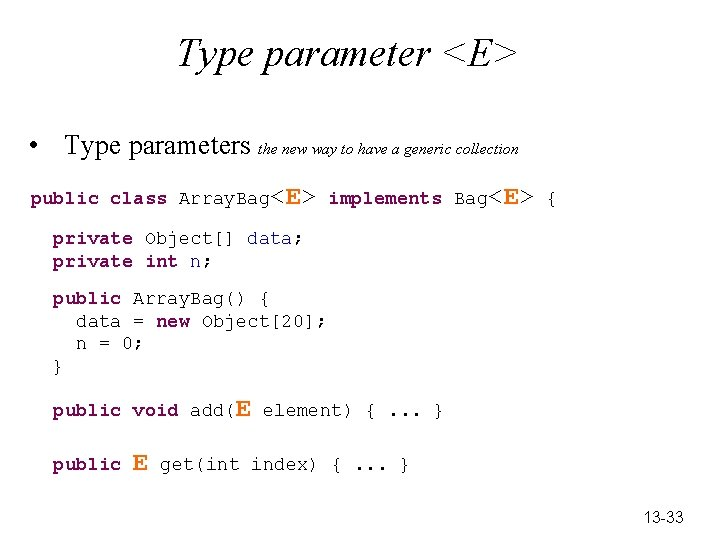Type parameter <E> • Type parameters the new way to have a generic collection public class Array. Bag<E> implements Bag<E> { private Object[] data; private int n; public Array. Bag() { data = new Object; n = 0; } public void add(E element) {. . . } public E get(int index) {. . . } 13 -33Can not have E[] • We can not declare arrays of a generic parameter public class Array. Bag<E> implements Bag<E> { private E[] data; public Array. Bag() { data = new E; ^ Cannot create a generic array of E 13 -34Can cast with (Type[]) • At runtime, the generic parameter E is really Object • We could use a cast like this: (E[]) public class Array. Bag<E> implements Bag<E> { private E[] data; public Array. List() { data = (E[]) (new Object); } 13 -35Use Object[] • Or we can use Object[] as the instance variable public class Array. Bag<E> implements Bag<E> { private Object[] data; public Array. Bag() { data = new Object; } 13 -36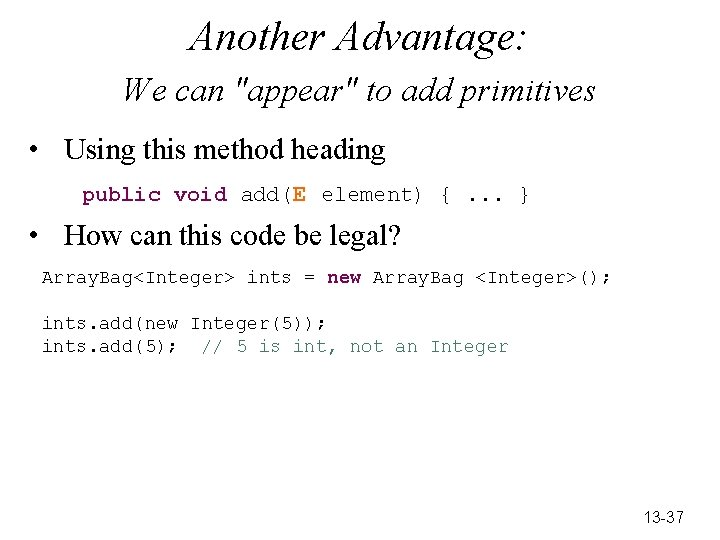Another Advantage: We can "appear" to add primitives • Using this method heading public void add(E element) {. . . } • How can this code be legal? Array. Bag<Integer> ints = new Array. Bag <Integer>(); ints. add(new Integer(5)); ints. add(5); // 5 is int, not an Integer 13 -37Primitives are appear to be Objects • To allow collections of primitive types, with Object[] Java provided wrapper classes: Integer Double Character Boolean Long Float These allow you to treat primitives as Objects (need new) • Before Java 5, wrapper objects can't handle arithmetic operators Integer an. Int = new Integer(50); int result = 2 * an. Int - 3; Error before Java 5 • Now objects appear as primitives and primitives as objects Integer i = 3; // assign int to Integer int j = new Integer(4); // Integer to int k = (3*new Integer(7)) + (4*i); // operators OK 13 -38How? Boxing / Unboxing • Autoboxing is the process of treating a primitive as if it were an reference type. When the compiler sees this Integer an. Int = 3; — the code transforms into this Integer an. Int = new Integer(3); • Java 1. 4 arithmetic requires int. Value or double. Value messages, for example: int answer = 2 * an. Int. int. Value(); • Java 5 allows this int answer = 2 * an. Int; 13 -39Collection Classes 13 -40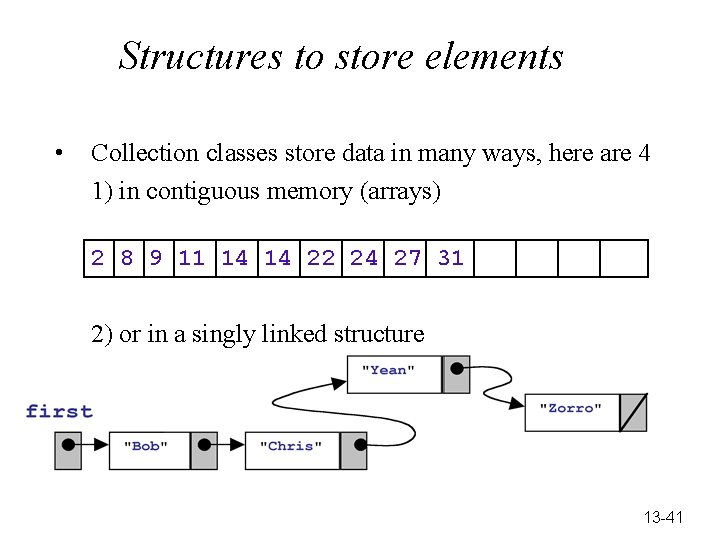Structures to store elements • Collection classes store data in many ways, here are 4 1) in contiguous memory (arrays) 2 8 9 11 14 14 22 24 27 31 2) or in a singly linked structure 13 -41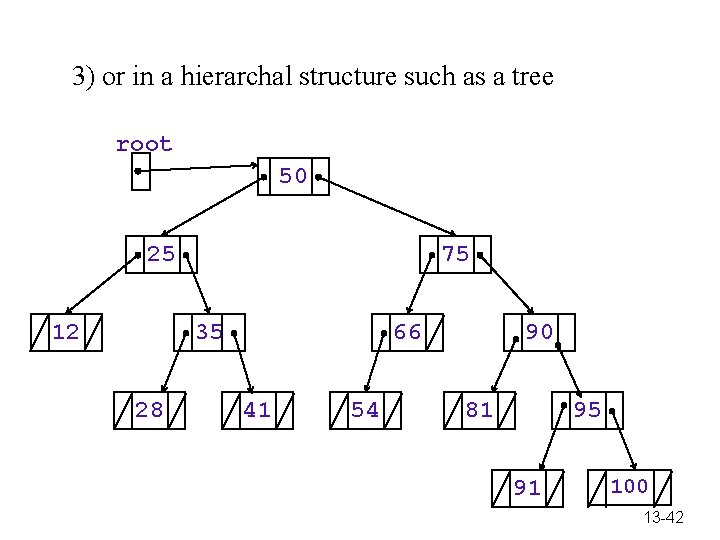3) or in a hierarchal structure such as a tree root 50 25 12 75 35 28 66 41 54 90 81 95 91 100 13 -424) or in hash tables • Maps associate a key with a value • e. g. your student ID and your student record • Elements could be stored in a hash table Array Index 0 Key Smith D Object (state is shown, which is the instance variables values of Employees Devon 40. 0 10. 50 1 'S' 1 null 2 Gupta C Chris 0. 0 13. 50 1 'S' 3 Herrs A Ali 20. 0 9. 50 0 'S' 4 null 5 Li X Xuxu 42. 5 12. 00 2 'M' 13 -43